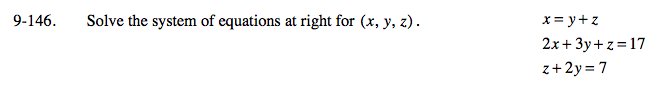### Home > A2C > Chapter 9 > Lesson 9.3.2 > Problem9-146

9-146.Substitute y + z for x into the second equaton.

2y + 2z + 3y + z = 17
3z + 5y = 17

Multiply the third equation by −3 then add it to the earlier equation.

3z + 5y = 17
−3z − 6y = −21

y = −4

Substitute 4 for y.

z + 8 = 7

Substitute 4 and −1 for y and z.

x = 4 − 1

y = 4

z = −1

x = 3

(3, 4, −1)